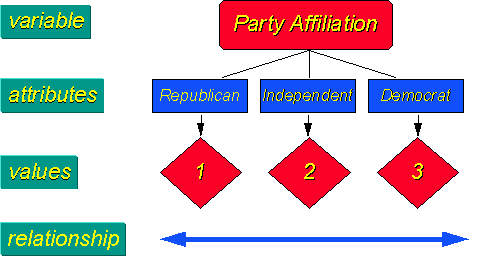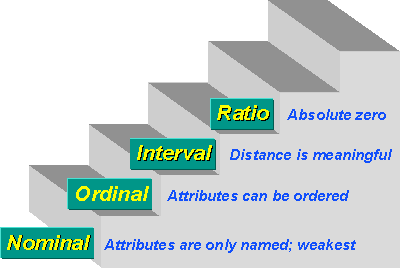Levels of Measurement

# Levels of Measurement

The level of measurement refers to the relationship among the values that are assigned to the attributes for a variable. What does that mean? Begin with the idea of the variable, in this example “party affiliation.”That variable has a number of attributes. Let’s assume that in this particular election context the only relevant attributes are “republican”, “democrat”, and “independent”. For purposes of analyzing the results of this variable, we arbitrarily assign the values `1`, `2` and `3` to the three attributes. The level of measurement describes the relationship among these three values. In this case, we simply are using the numbers as shorter placeholders for the lengthier text terms. We don’t assume that higher values mean “more” of something and lower numbers signify “less”. We don’t assume the the value of `2` means that democrats are twice something that republicans are. We don’t assume that republicans are in first place or have the highest priority just because they have the value of `1`. In this case, we only use the values as a shorter name for the attribute. Here, we would describe the level of measurement as “nominal”.

## Why is Level of Measurement Important?

First, knowing the level of measurement helps you decide how to interpret the data from that variable. When you know that a measure is nominal (like the one just described), then you know that the numerical values are just short codes for the longer names. Second, knowing the level of measurement helps you decide what statistical analysis is appropriate on the values that were assigned. If a measure is nominal, then you know that you would never average the data values or do a t-test on the data.

There are typically four levels of measurement that are defined:

• Nominal
• Ordinal
• Interval
• Ratio

In nominal measurement the numerical values just “name” the attribute uniquely. No ordering of the cases is implied. For example, jersey numbers in basketball are measures at the nominal level. A player with number `30` is not more of anything than a player with number `15`, and is certainly not twice whatever number `15` is.

In ordinal measurement the attributes can be rank-ordered. Here, distances between attributes do not have any meaning. For example, on a survey you might code Educational Attainment as 0=less than high school; 1=some high school.; 2=high school degree; 3=some college; 4=college degree; 5=post college. In this measure, higher numbers mean more education. But is distance from 0 to 1 same as 3 to 4? Of course not. The interval between values is not interpretable in an ordinal measure.In interval measurement the distance between attributes does have meaning. For example, when we measure temperature (in Fahrenheit), the distance from 30-40 is same as distance from 70-80. The interval between values is interpretable. Because of this, it makes sense to compute an average of an interval variable, where it doesn’t make sense to do so for ordinal scales. But note that in interval measurement ratios don’t make any sense - 80 degrees is not twice as hot as 40 degrees (although the attribute value is twice as large).

Finally, in ratio measurement there is always an absolute zero that is meaningful. This means that you can construct a meaningful fraction (or ratio) with a ratio variable. Weight is a ratio variable. In applied social research most “count” variables are ratio, for example, the number of clients in past six months. Why? Because you can have zero clients and because it is meaningful to say that “…we had twice as many clients in the past six months as we did in the previous six months.”

It’s important to recognize that there is a hierarchy implied in the level of measurement idea. At lower levels of measurement, assumptions tend to be less restrictive and data analyses tend to be less sensitive. At each level up the hierarchy, the current level includes all of the qualities of the one below it and adds something new. In general, it is desirable to have a higher level of measurement (e.g., interval or ratio) rather than a lower one (nominal or ordinal).